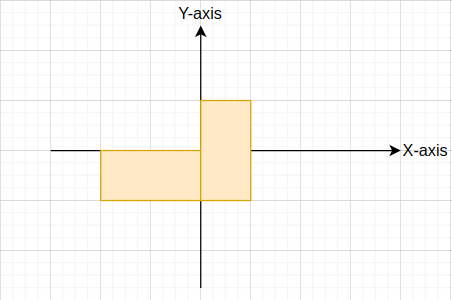# Total area of overlapping rectangles

Posted: 10 Nov, 2020
Difficulty: Easy

## PROBLEM STATEMENT

#### You are given two arbitrary rectangles on a 2-D coordinate plane, which may have an intersecting area. You have to find the net area covered by both the rectangles on the cartesian plane.#### Note:

``````1. For a rectangle, its top left and bottom right coordinates are given.

2. Coordinates of the rectangles are integer values.

3. Edges of the given rectangles will always be parallel to the X and Y coordinate axes of the cartesian plane.

4. It is guaranteed that both the rectangles will have at least a unit area.
``````
##### Input Format:
``````The first line of the input contains an integer 'T' denoting the number of test cases.

The first line of each test case contains 4 space-separated integer values 'x1', 'y1', 'x2', 'y2' denoting the top left ('x1', 'y1') and bottom-right ('x2', 'y2') coordinates of the first rectangle.

The second line of each test case contains 4 space-separated integer values 'x3', 'y3', 'x4', 'y4' denoting the top left ('x3', 'y3') and bottom-right ('x4', 'y4') coordinates of the second rectangle.
``````
##### Output Format:
``````For each test case, return an integer denoting the net area of two rectangles.
``````
##### Note:
``````You do not need to print anything, it has already been taken care of. Just implement the given function.
``````
##### Constraints:
``````1 <= T <= 10^5
-10^9 <= x1, y1, x2, y2 <= 10^9
x1 < x2, x3 < x4
y1 > y2, y3 > y4

Time Limit: 1sec
``````Approach 1
• The problem is essentially two questions:
1. How to know if the two rectangles have an intersecting area? Because if they do have some common area we need to subtract it from our final answer.
2. If the two rectangles have a common area, how to find it?

• Let’s consider two rectangles rectangle1 and rectangle2. Where left-most top point and right-most bottom point of rectangle1 are ('x1', ‘y1’), ('x2', ‘y2’). And the same for rectangle2 are ('x3', ‘y3’), ('x4', ‘y4’).
• To know if two rectangles have some common area, we need to consider only four situations: ‘x1’ >= ‘x4’ or ‘y1’ <= ‘y4’ or ‘x2’ <= ‘x3' or ‘y2’ >= ‘y3’. In such cases, rectangle ('x1', ‘y1’, ‘x2’, ‘y2’) will not overlap with the rectangle ('x3', ‘y3’, ‘x4’, ‘y4’).
• To calculate the common area, we only need to know the coordinates of the bottom left corner and top right corner of the overlapped area.
• In any case we only need to know max('x1', ‘x3’), min('x2', ‘x4’), max('y1', ‘y3’) and min('y2', ‘y4’)  to calculate the overlapped area.
• Net area = Area of rectangle 1 + Area of rectangle 2 - Intersection or Overlapped area.